# Using DATE function in Excel - formula examples to calculate dates

When it comes to calculating dates in Excel, DATE is the most essential function to understand. As you probably know, Excel does not keep the year, month and day for a date, nor does it explicitly store weekday information in a cell. Instead, Microsoft Excel stores dates as serial numbers and this is the main source of confusion.

Not all Excel date functions can recognize dates entered as text values, therefore it's not recommended to supply dates directly in calculations. Instead, you should use the DATE function to get a serial number representing the date, the number that Excel understands and can operate on.

## Excel DATE function syntax

What the Excel DATE function does is return the serial number of a specified date. It has the following arguments:

DATE(year, month, day)

Year - represents the year of the date. Excel interprets the year argument according to the date system set up on your computer. By default, Microsoft Excel for Windows uses the 1900 system. In this date system:

• If year is between 1900 and 9999 inclusive, exactly that value is used for the year. For example, `=DATE(2015, 12, 31)` returns December 31, 2015.
• If the year argument is between 0 and 1899 inclusive, Excel calculates the year by adding the specified number to 1900. For example, `=DATE(100, 12, 31)` returns December 31, 2000 (1900 + 100).
• If year is less than 0 or greater than 9999, a DATE formula will return the #NUM! error.
Tip. To avoid confusion, always supply four digit years. For example, if you input "01" or "1" in the year argument, your DATE formula will return the year of 1901.

Month - an integer representing the month of the year, from 1 (January) to 12 (December).

• If month is greater than 12, Excel adds that number to the first month in the specified year. For example, `=DATE(2015, 15, 5)` returns the serial number representing March 1, 2016 (January 5, 2015 plus 15 months).
• If month is less than 1 (zero or negative value), Excel subtracts the magnitude of that number of months, plus 1, from the first month in the specified year. For example, `=DATE(2015, -5, 1)` returns the serial number representing July 1, 2014 (January 1, 2015 minus 6 months).

Day - an integer corresponding to the day of the month, from 1 to 31.

As well as month, the day argument can be supplied as a positive and negative number, and Excel calculates its value based on the same principles as described above.

Tip. At first sight, supplying negative values in the `month` or `day` argument of the Excel DATE function may seem absurd, but in practice it may turn out quite useful, for example in the complex formula that converts a week number to a date.

The DATE function is available in all versions of Excel 2013, Excel 2010, Excel 2007, 2003, XP, 2000 as well as in Excel 2016.

## Excel DATE formula examples

Below you will find a few examples of using DATE formulas in Excel beginning with the simplest ones.

#### Example 1. A simple DATE formula to return a serial number for a date

This is the most obvious use of the DATE function in Excel:

`=DATE(2015, 5, 20)` - returns a serial number corresponding to 20-May-2015.

Instead of specifying the values representing the year, month and day directly in a date formula, you can get some or all arguments as results of other Excel date functions:

`=DATE(YEAR(TODAY()), 1, 1)` - returns the serial number for the first day of the current year.

`=DATE(YEAR(TODAY()), MONTH(TODAY(), 1)` - returns the serial number for the first day of the current month in the current year.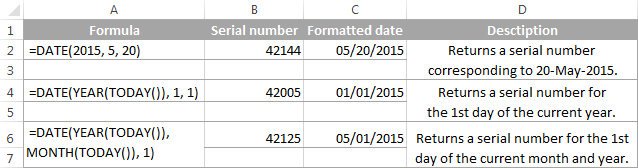#### Example 2. Excel DATE formula to return a date based on values in other cells

The DATE function is very helpful for calculating dates where the year, month, and day values are stored in other cells. For example:

`=DATE(A2, A3, A4)` - returns the serial number for the date, taking the values in cells A2, A3 and A4 as the year, month and day arguments, respectively.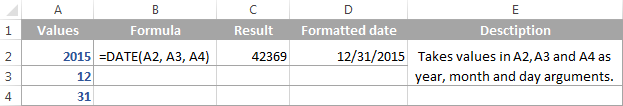#### Example 3. DATE formula to convert a text string to a date

Another scenario when the Excel DATE function proves useful is when the dates are stored in the format that Microsoft Excel does not recognize, for instance DDMMYYYY. In this case, you can use DATE in liaison with other functions to convert a date stored as a text string into a serial number representing the date:

`=DATE(RIGHT(A2,4), MID(A2,3,2), LEFT(A2,2))`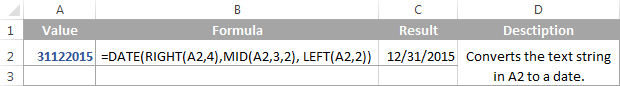#### Example 4. Adding and subtracting dates in Excel

As already mentioned, Microsoft Excel stores dates as serial numbers and operates on those numbers in formulas and calculations. That is why when you want to add or subtract some days to/from a given date, you need to convert that date to a serial number first by using the Excel DATE function. For example:

• Adding days to a date:

`=DATE(2015, 5, 20) + 15`

The formula adds 15 days to May 20, 2015 and returns June 4, 2015.

• Subtracting days from a date:

`=DATE(2015, 5, 20) - 15`

The result of the above formula is May 5, 2015, which is May 20, 2015 minus 15 days.

• To subtract a date from today's date:

`=TODAY()-DATE(2015,5,20)`

The formula calculates how many days are between the current date and some other date that you specify.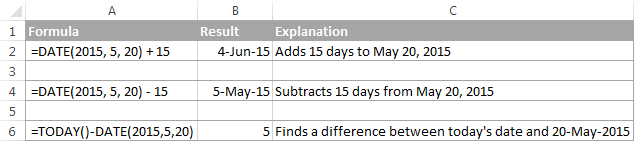If you are adding or subtracting two dates that are stored in some cells, then the formula is as simple as =A1+B1 or A1-B1, respectively.

And here are a few more examples where Excel DATE is used in combination with other functions in more complex formulas:

## Using Excel DATE formulas in conditional formatting

In case you want not only to calculate but also highlight dates in your Excel worksheets, then create conditional formatting rules based on DATE formulas.

Supposing you have a list of dates in column A and you want to shade dates that occurred earlier than 1-May-2015 in orange and those that occur after 31-May-2015 in green.

The DATE formulas you want are as follows:

Orange: `=\$A2<DATE(2015, 5, 1)` - highlights dates less than 1-May-2015

Green: `=\$A2>DATE(2015, 5, 31)` - highlights dates greater than 31-May-2015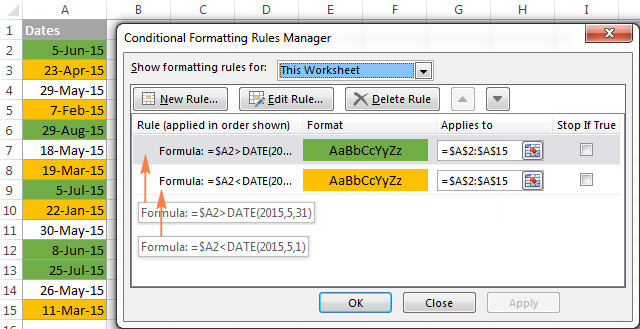For the detailed steps and more formula examples, please see How to conditionally format dates in Excel.

## How to make date formulas in Excel with Date & Time Wizard

Though DATE is the main function to work with dates in Excel, a handful of other functions are available to tackle more specific tasks. You can find the links to in-depth tutorials at the end of this article.

Meanwhile, I'd like to present you our Date & Time Wizard - a quick and easy way to calculate dates in Excel. The beauty of this tool is that outputs the results as formulas, not values. Thus you have a kind of 'two birds, one stone' opportunity - get the result faster and learn Excel date functions along the way :)

The wizard can perform the following calculations:

• Add years, months, weeks, days, hours, minutes and seconds to the specified date.
• Subtract years, months, weeks, days, hours, minutes and seconds from the specified date.
• Calculate the difference between two dates.
• Get age from the birthdate.

For example, here's how you can add 4 different units in cells B3:E3 to the date in A4. The formula in B4 is built in real-time as you change the conditions: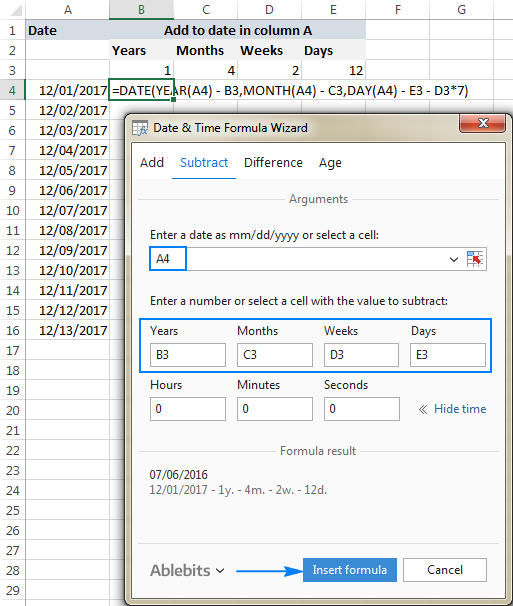If you are curious to explore other capabilities of the wizard, feel free to download a 14-day trial version of the Ultimate Suite which includes this as well as 60 more time saving add-ins for Excel.

I thank you for reading and hope to see you on our blog next week!

-->

### 418 Responses to "Using DATE function in Excel - formula examples to calculate dates"

1.Flamnanda says:

Hi,
I am trying to create a spreadsheet that alerts me to the approaching time limit of some applications.
I need a formula that will use the "valid date" in column A and "decision date" in column E to tell me via colour code:

* Red - 0-7 days from valid date to decision date
* Amber - 8-24 days from valid date to decision date
* Green - 25-100 days from valid date to decision date

I've tried a few variations of things with no luck and it's driving me insane!!

2.matin says:

My completion date should not chnage from previous date to current date. i want a fromula to track how many docs an executive has completed yday, today and so on..I have used now fromula as well but it chnages the date to present.

3.VPS says:

4.DavidDiulk says:

Thank you for the info!

5.Maneo says:

Hi
I need a formula to calculate employees leave days "to date"

6.Beth S. Lagangan says:

How would I separate birthday column of 07/06/48 to a separate column of month, day, year?
Thank you.

7.Beth S. Lagangan says:

separate 07/04/1959 to 07, 04, 1959 per column

8.Matt Weiss says:

I wanted to create an Excel formula that produced an 18th birthday based on a date. Through trial and error, I came up with this one.
Cell A1 = 4/26/2008
Cell B1: =DATE(YEAR(A1)+DATEDIF(A1,TODAY(),"y")+(18-ROUNDDOWN(((TODAY()-A1)/365),0)),MONTH(A1),DAY(A1))
Result = 4/26/2026

9.AJ says:

I am trying to create a formula to find the departure date from a loading date...for eg if loading date is 2nd December and departure for that specific country is Tuesday/ Friday then the departure date should be displayed as 3rd December. if loading date is 4th December then departure date should be 6th December Friday. I have 1 column with the country which is vlook'd up from another sheet in the workbook along with loading dates

10.Cheryl says:

I am trying to create a schedule in table format where the date format is ex (Sunday, Dec 02)and want it to autofill across the top. I also need this to be able search by day or date for an app.

11.Steve says:

Hi Can you help.
I am trying to track absence and using =DATEDIF(D62,\$N\$2,"d") to give me the total of days someone if off sick, however I need it to stop counting when they return to work. Any ideas?
Thanks,
Steve

12.Runee says:

I have a question.
Im new to excel and my boss wants me to calculate Day,month and year from a different cell.
and it's about retiredate. I have to calculate from their birthdate to the next 60 years. I mean I can use this formulas [=date(year(cell)+60+if(month(cell)>=10,1,0),9,30)] but just in case their birthdate is in the same cell. but from the different cell I can't. I really don't know what to do with this. I really appreciate if someone can help me.
***sorry for my english***

13.Raj says:

Hi
i am trying to get a yes or no answer for:
Employee's hire date is greater than 3-31-2019, yes or no
but an employee hired on 1-2-1990 is showing as "no"

14.Rashid Karani says:

I WOULD LIKE TO DRAFT AGEING REPORT USING EXCEL.
WE PROVIDE LOANS, I WOULD MY EXCEL TO HIGHLIGHT A DAY BEFORE THE DUE DATE

15.Ella says:

Hello
I am trying to create a table whereby I set one anticipated start date and this can then autofill other cells by putting in how many weeks after (eg. start date is 05.03.20 so if I put a 2 in the weeks column the date would show up as 19.03.20). Does anyone know if this is possible/how to do it?
Many thanks
Ella.

16.Headtrix says:

How can I from say cell N11 Up until I11 count all the days that art between as a total amount of days that have passed so far does my question make sense?

I have a start date and a end date but as the days in between get added in as time goes on they need to be subtracted from the amount of days in my days *total column*.

*Start* *End* *Total Days for project*
03/03/2020 dates dates dates 05/05/2020 Days passed/left?

60+ professional tools for Excel